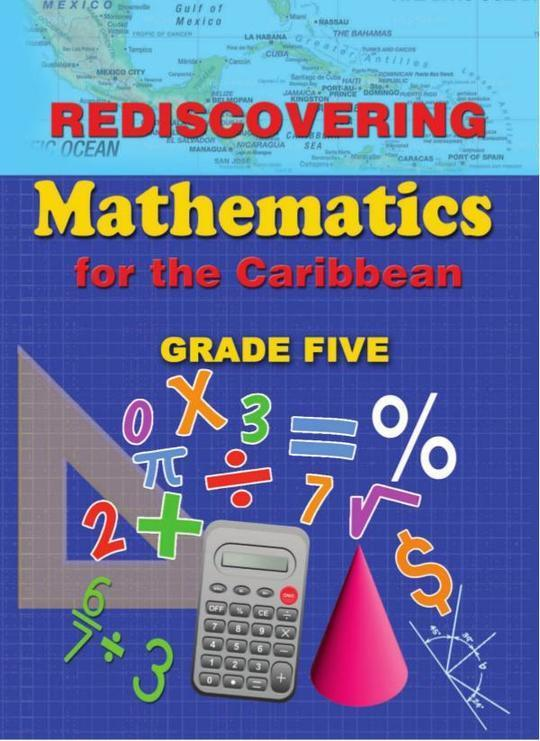\$9.99

# Rediscovering Mathematics for the Caribbean Grade Five

By Dr. Adrian Mandara
US\$ 9.99
The publisher has enabled DRM protection, which means that you need to use the BookFusion iOS, Android or Web app to read this eBook. This eBook cannot be used outside of the BookFusion platform.
Book Description

Rediscovering Mathematics for the Caribbean: Grade Five, is the seventh of a comprehensive series of eight textbooks which covers the requirements of the Mathematics syllabuses for Primary Schools in the Caribbean. The text provides a firm foundation in Mathematics for primary school pupils utilizing a learner-centred approach.

Some Key Features:

• Basic numeracy skills are emphasized and topics are carefully introduced.

• Numerals, exercises and illustrations provide pupils with a lot of practice, thereby enhancing their understanding of mathematical concepts.

• Concepts are expressed in simple, clear and easy to understand language that is appropriate to the grade level and subject.

• Meaningful connections are made to real life situations.

Comprehensive worked examples act as step-by-step guides for pupils who have difficulty problem-solving. These numerous worked example at the Grades 5 and 6 levels are clearly presented and should provide much needed guidance to pupils.

Many teaching tips are included to guide pupils through different problems.

The “Think About It” sections are filled with questions that extend knowledge and help to develop pupils’ critical thinking and problem-solving skills.

• Cover Page
• Title Page
• Acknowledgement
• Message to Student
• Dedication
• Contents
• Assess Yourself
• Sets
• Notation and Place Value
• Kinds of Numbers
• Notation
• Introducing the Million
• Numerals and Number Names
• Number Patterns
• Place Value
• Expanded Notation
• Powers of 10 (exponents)
• The Digit Zero
• Cardinal and Ordinal Numbers
• Sequencing
• Rounding Whole Numbers?
• Roman Numerals
• Using the Calculator
• Revision
• Properties of Addition and Multiplication
• Subtraction
• Revision
• Multiplication
• Multiplication of 3 Numbers
• Multiplication by Multiples of 10
• Division
• Division facts – Divisibility
• Think About It
• Order of Operations
• Factors and Multiples
• What are Factors?
• Prime and Composite Numbers
• Common Factors
• Prime Factorization
• Lowest Common Multiple (L.C.M.)
• Highest Common Factor (H.C.F.)
• Square and Square Root of Numbers
• Square of Numbers
• Square Roots
• Fractions
• What are Fractions?
• Comparing Fractional Parts
• Equivalent Fractions
• Changing Improper Fractions to Whole Numbers or Mixed Numbers
• Addition and Subtraction of Like Fractions
• Addition and Subtraction of Related Fractions
• Adding Unlike Fractions
• Subtracting Unlike Fractions
• Adding Fractions and Whole Numbers
• Multiplying Fractions by Whole Numbers
• Multiplying Whole Numbers by Fractions
• Changing Mixed Numbers to Improper Fractions
• Multiplying a Mixed Number by a Whole Number
• Multiplying a Whole Number by a Mixed Number
• Multiplying a Fraction by a Fraction
• Division of Fractions
• Dividing Mixed Numbers
• Fractional Parts of a Quantity
• Think About It
• Decimals
• Tenths
• Hundredths
• Thousandths
• Fractions and decimals
• Writing Fractions as Decimals
• Writing Decimals as Fractions
• Addition and Subtraction of Decimal Fractions
• Multiplying and Dividing by 10, 100, and by 1,000
• Multiplying Decimals by Decimals
• Comparing and Ordering Decimals
• Think About It
• Percentage
• Fractions, Decimals and Percentages
• Using Percentages
• Expressing as a Percentage
• Increment and Discount
• Direct Proportion
• Estimation And Approximation
• Money
• Rates of Exchange
• Profit and Loss
• Percentage Profit and Loss
• Transactions Involving Money
• Banking
• Financial Institutions
• Value Added Tax
• Hire Purchase
• Think About It
• Measurement
• LENGTH
• Perimeter
• Area
• The Area of a Square
• The Area of a Triangle
• MASS
• Volume
• CAPACITY
• TEMPERATURE
• TIME
• Timeline
• THE CALENDAR
• SCALE DRAWING
• THINK ABOUT IT
• Geometry
• Geometric Ideas
• Point, Plane, Lines
• Kinds of Lines
• Measurement of Straight Lines
• Measurement of Curved Lines
• Geometrical Solids
• What is a Solid?
• Some Common Solids
• Solids and Their Nets
• Triangles
• Basic properties of triangles
• Properties of Perpendicular and Parallel Lines
• Symmetry
• Congruence
• Angles & The Use of Protractors
• Definition of an Angle
• The Protractor
• Kinds of Angles
• Constructing Angles
• Use of Set Square
• Drawing Perpendicular Lines
• Drawing Parallel Lines
• Polygons
• Tiling and Tessellation
• Uses of The Compasses
• Constructing circles
• Bisecting Straight Lines
• Circumference and Area of a Circle
• The Compass And Direction
• Algebra
• Use of Letters to Represent Numbers
• Mathematical Sentences
• Addition and Subtraction Sentences
• Multiplication and Division Sentences
• Open Sentence
• Data Handling, Statistics & Probability
• Data Handling
• Graphical Representation of Data
• What is Statistical Data?
• The Different Types of Graphs
• Research/Study
• The Survey
• Interpreting Data
• Mean
• Median
• Mode
• Range
• Probability
• Assessment
• Assessment
• Back Cover
The book hasn't received reviews yet.
You May Also Like